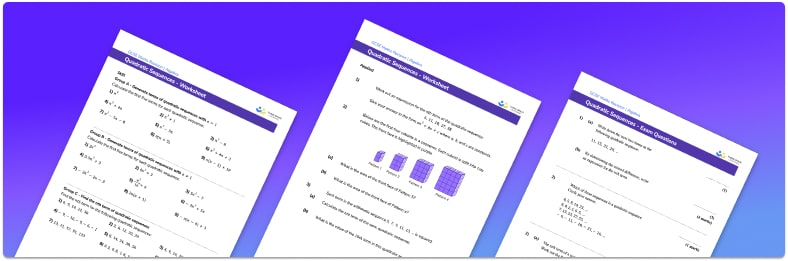• This field is for validation purposes and should be left unchanged.

You can unsubscribe at any time (each email we send will contain an easy way to unsubscribe). To find out more about how we use your data, see our privacy policy.

• Section 1 of the quadratic sequences worksheet contains 27 skills-based questions, in 3 groups to support differentiation
• Section 2 contains 3 applied questions with a mix of worded problems and deeper problem solving questions
• Section 3 contains 5 higher level GCSE exam style questions to practise working with quadratic sequences, including finding the nth term of the sequence
• Answers and a mark scheme for all questions are provided
• Questions follow variation theory with plenty of opportunities for students to work independently at their own level
• All questions created by fully qualified expert secondary maths teachers
• Suitable for GCSE maths revision for AQA, OCR and Edexcel exam boards

### Quadratics sequences at a glance

Quadratic sequences are number sequences based on the square numbers. The differences between the terms increase or decrease by the same amount; this is called the second difference between the terms. Quadratic sequences tend to involve integers rather than decimals.

Quadratic sequences have an nth term formula which can be used to generate terms of the number sequence. The nth term rule can be used to find any missing terms in a quadratic sequence, similar to how the nth term of a linear sequence can be used.

The nth term of a quadratic sequence can be found by finding the first differences, and then working out the second differences. The second differences should all be the same. We then need to divide the second difference by 2 in order to work out the coefficient of the squared term of the nth term of this quadratic sequence. We can then compare this quadratic part of the sequence to the original sequence to create a separate linear sequence. The quadratic part and the linear part then combine to give the overall nth term of the quadratic sequence.

Looking forward, students can then progress to additional algebra worksheets, for example a solving equations with fractions worksheet or a simultaneous equations worksheet.For more teaching and learning support on Algebra our GCSE maths lessons provide step by step support for all GCSE maths concepts.

## Related worksheets

Arithmetic Sequence Worksheet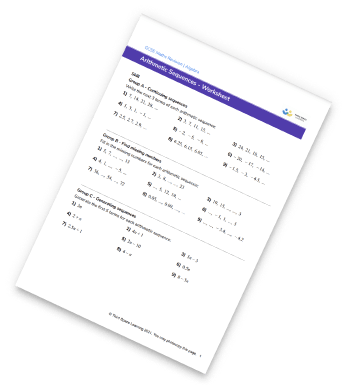Nth Term Worksheet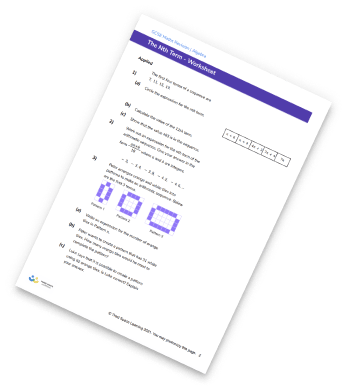Sequences Worksheet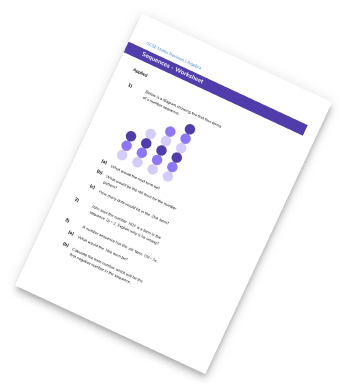Geometric Sequence Worksheet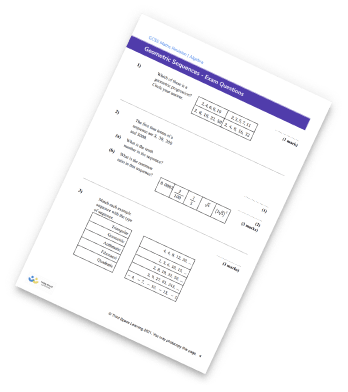## Do you have KS4 students who need more focused attention to succeed at GCSE?There will be students in your class who require individual attention to help them succeed in their maths GCSEs. In a class of 30, it’s not always easy to provide.

Help your students feel confident with exam-style questions and the strategies they’ll need to answer them correctly with our dedicated GCSE maths revision programme.

Lessons are selected to provide support where each student needs it most, and specially-trained GCSE maths tutors adapt the pitch and pace of each lesson. This ensures a personalised revision programme that raises grades and boosts confidence.

Find out more